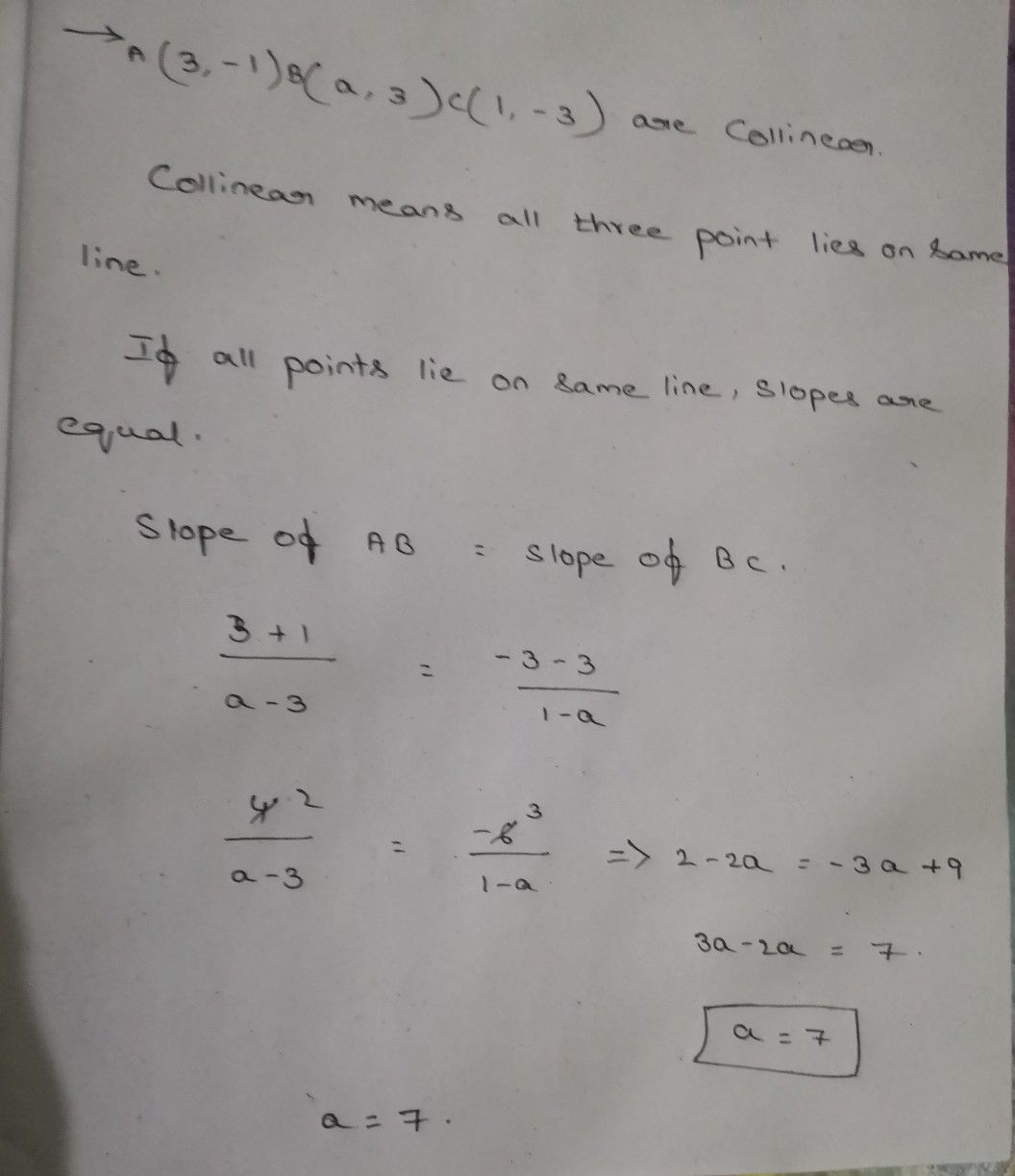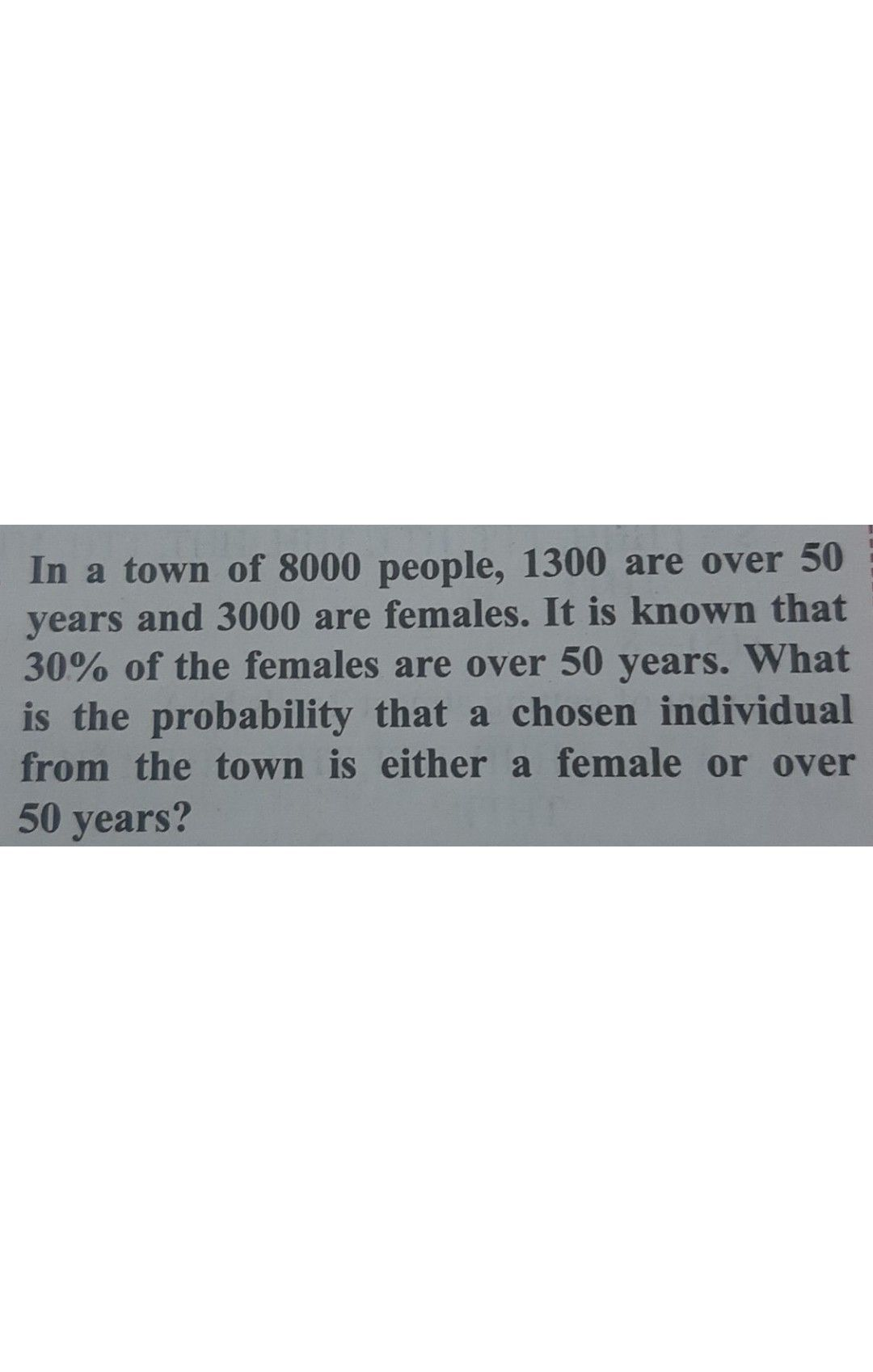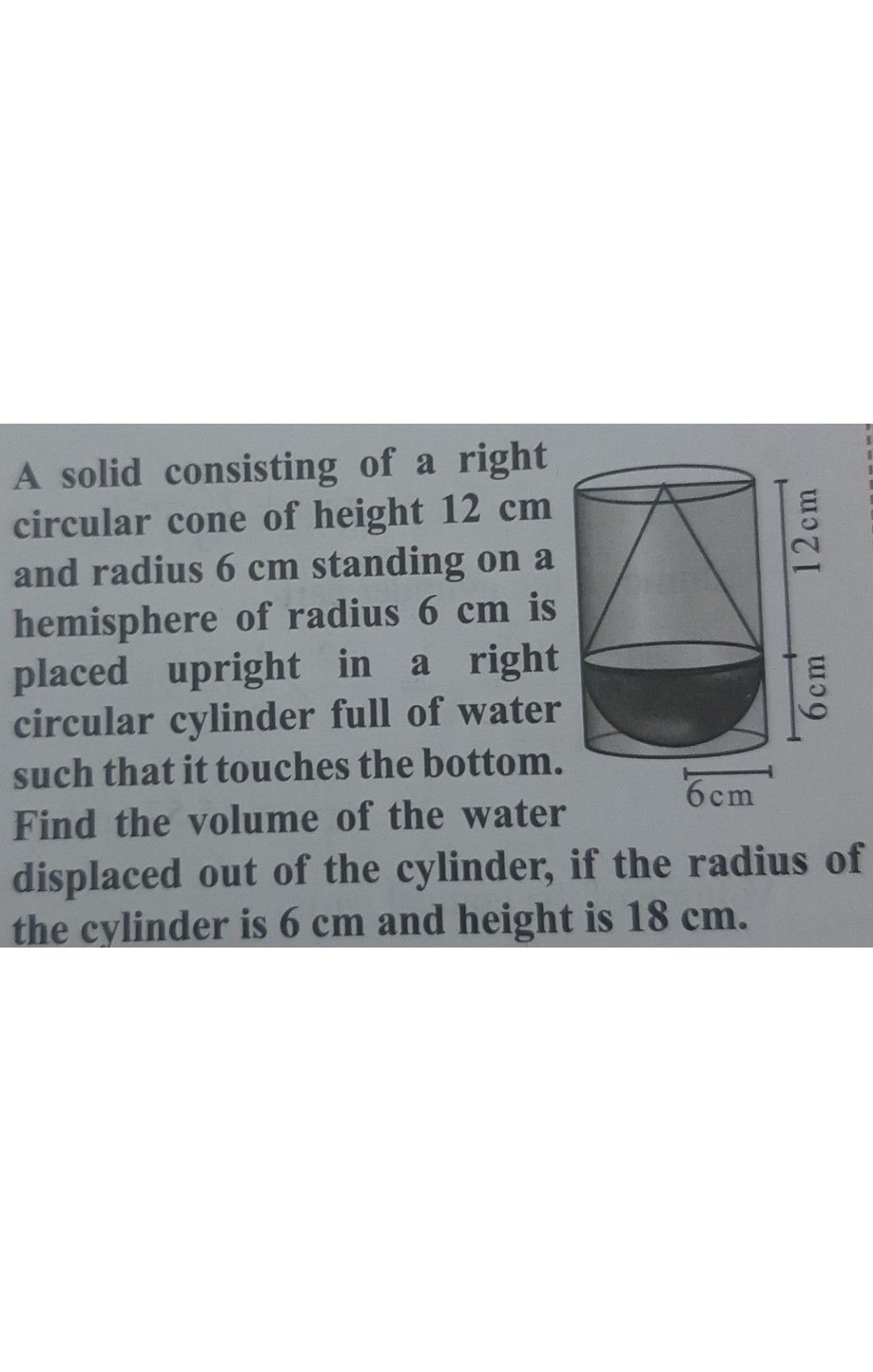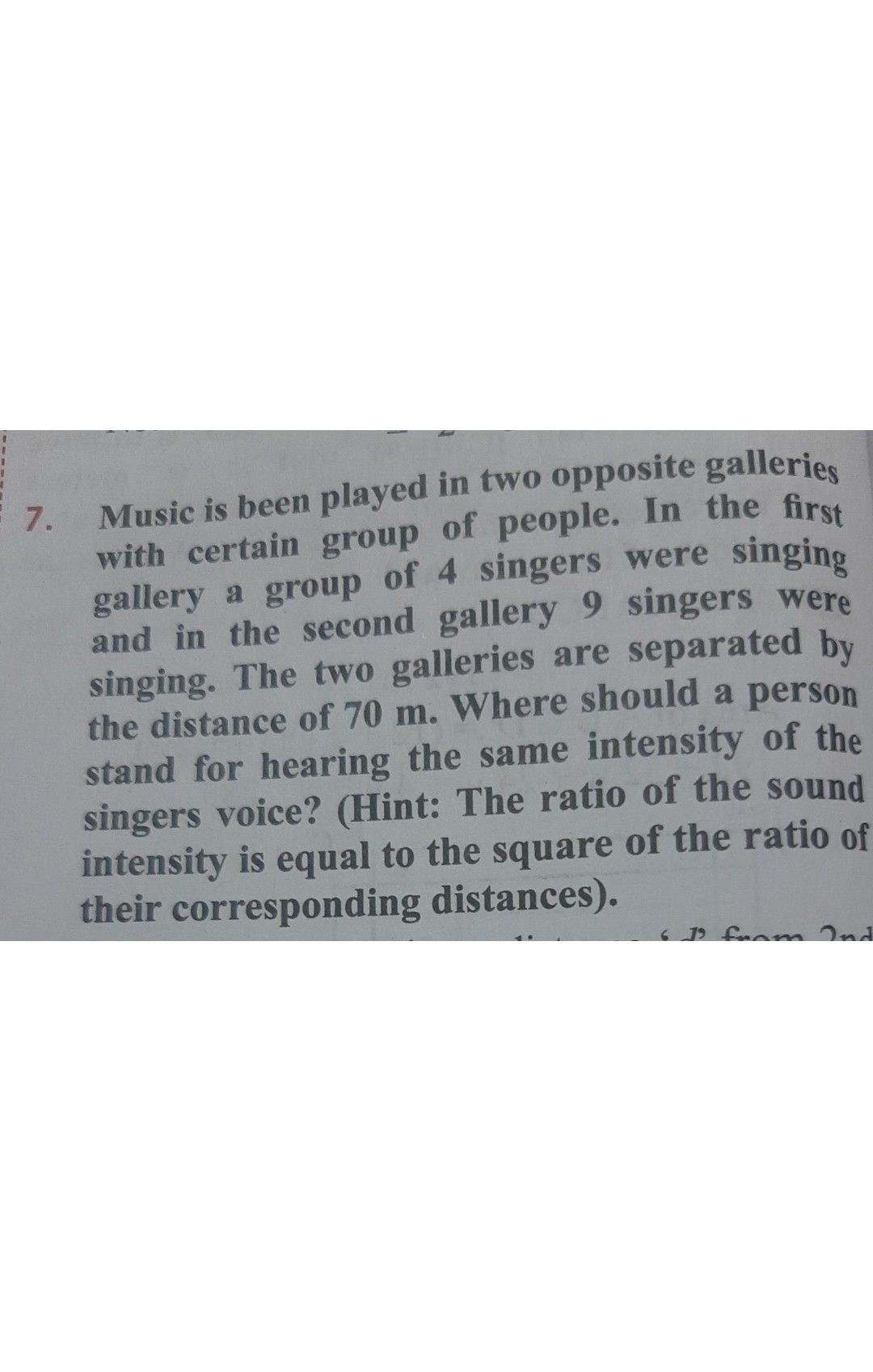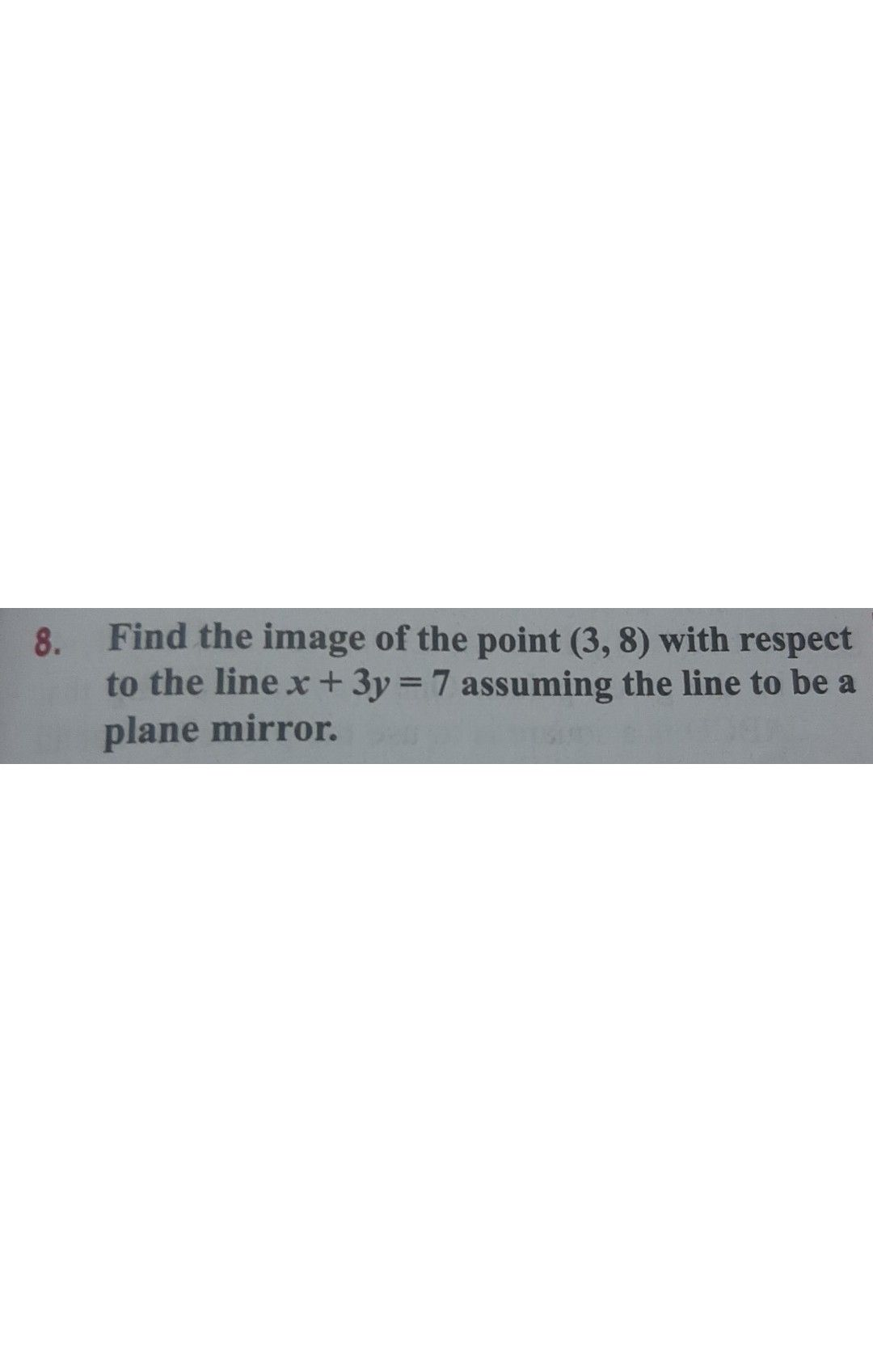Symbol
ProblemIf the three points $\left(3,-1\right),\left(a,3\right),\left(1,-3\right)$ are collinear, find the value of $a.$